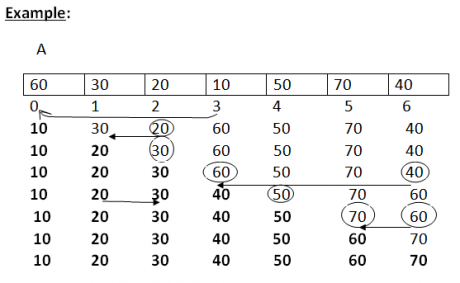Selection sort in Data Structures
Ratings:
(4)
Views:1079## Selection sort

Suppose an array A with ‘n’ elements A,A,A….. is in memory. The selection sort algorithm in data structures for sorting ‘A’ works as follows Pass 1:  First find the smallest element in the list and put it into the first position. Then find the second smallest element in the list of ‘N’ elements A,A,A------ and then interchange A[Loc] and A is sorted. Pass 2: Find the location LOC of the smallest in the sub list of n-1 elements A,A,A then A,A sorted Pass N-1: Find the location LOC of the smaller of the elements A[N-1], A[N] and then interchange A[LOC] and A[N-1] then : A,A,A-----  is sorted.### Selection Sort Program

Implementation of selection sort # include<stdio.h> void selection sort(int a[],int n); void main() { int a,n,i; clrscr(); printf(“Enter n value \n”); scanf(“%d”,&n); printf(“Enter those numbers \n”); for (i=0;i<n;i++) { scanf(“%d”,&a[i]); } selection sort(a,n); printf(“Following are the elements in sorting orger: \n”); for(i=0;i<n;i++) { printf(“%5d”,a[i]); } } void selection sort(int a[], int n) { int i, min Loc, t,j; for(i=0;i<n-1;i++) { MinLOC=i; for(j=i+1; j<n;j++) { if(a[j]<a[minLoc]) minLoc=j; } t=a[i]; a[i]=a[MinLoc]; a[MinLoc]=t; } }

### Time complexity :

Complexity of the selection sort algorithm f(n)=(n-1)+(n-2)+-------- +2+1=n(n-1)/2=0(n2)

 Algorithm Worst cape Average cape selection sort n(n-1)/2=o(n2) n(n-1)/2=o(n2)

### Output:

Enter n value : 7 Enter those numbers : 60     30     20     10     50     70     40   Following are the elements in the sorting order: 10     20     30     40     50     60     70